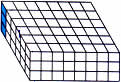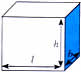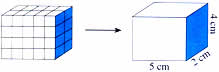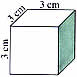Thursday , July 7 2022# NCERT 5th Class (CBSE) Mathematics: Area And Volume

Step 2: Find the number of layers. This is the same as the height in cm.

Height = 3 cubesStep 3: Multiply the result from step 1 with the height to get the total number of cm cubes48 × 3 = 144 cubes

Volume of the box is 144 cu. cm

Cube per layer             ×           Number of layers      =   Volume

length × breadth      ×          height                =     Volume

8 cm     ×     6 cm        ×       3 cm      =     144 cm3

Volume = length × breadth × height ← Formula for volume

Examples

(a) Find the volume of this cuboid or rectangular solid.Volume = × × h

= 5 cm × 2 cm × 4 cm = 40 cm3

(b) A cube is special cuboid with all sides equal. Find the volume of this cube.Volume = side × side × side

= 3 cm × 3 cm × 3 cm

= 27 cu. cm.

(c) Find the height of a box with volume 84 cu. cm, if the length and breadth are 4 cm and 3 cm, respectively.

Length = 4 cm, breadth = 3 cm, volume = 84 cu. cm, height = ?

volume = l × b × h

h = v/× b

h = 84/4 × 3 = 84/12 = 7

h = 7 cm

Check: 4 cm × 3 cm ×  7 cm = 84 cu. cm

(d) l = o.5 m, b = 15 cm, h = 9 cm, V = ?

Change m to cm.

0.5 m = 50 cm

Volume = 50 cm × 15 cm × 9 cm = 6750 cu. cm

## चुनौती हिमालय की 5th NCERT CBSE Hindi Rimjhim Ch 18

चुनौती हिमालय की 5th Class NCERT CBSE Hindi Book Rimjhim Chapter 18 प्रश्न: लद्दाख जम्मू-कश्मीर राज्य में …